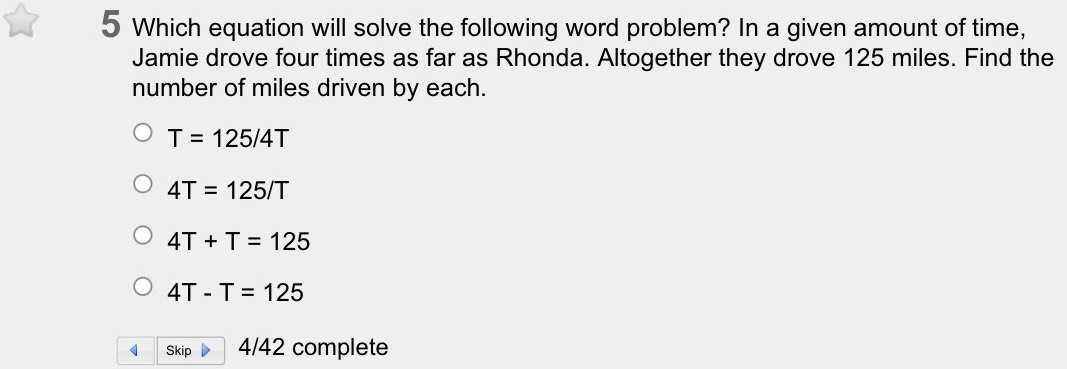### ¿Todavía tienes preguntas de matemáticas?

Pregunte a nuestros tutores expertos
Algebra
Pregunta$$5$$ Which equation will solve the following word problem? In a given amount of time, Jamie drove four times as far as Rhonda. Altogether they drove $$125$$ miles. Find the number of miles driven by each.

$$\bigcirc T = 125 / 4 T$$

$$\bigcirc 4 T = 125 / T$$

$$\bigcirc 4 T + T = 125$$

$$\bigcirc 4 T - T = 125$$